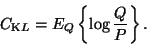Next: Model structure Up: Bayesian learning Previous: Parametric approximation of the

## Ensemble learning

Ensemble learning [3,6], also known as variational learning, is a recently developed method for parametric approximation of posterior pdfs where the search takes into account the probability mass of the models. Therefore, it does not suffer from overlearning. The basic idea is to minimise the misfit between the posterior pdf and its parametric approximation.

Let P denote the exact posterior pdf and Q its parametric approximation. The misfit is measured with the Kullback-Leibler divergence between P and Q and thus the cost function CKL is(1)

Notice that the Kullback-Leibler divergence involves an expectation over a distribution and, consequently, is sensitive to probability mass rather than probability density.

Harri Lappalainen
1999-05-25